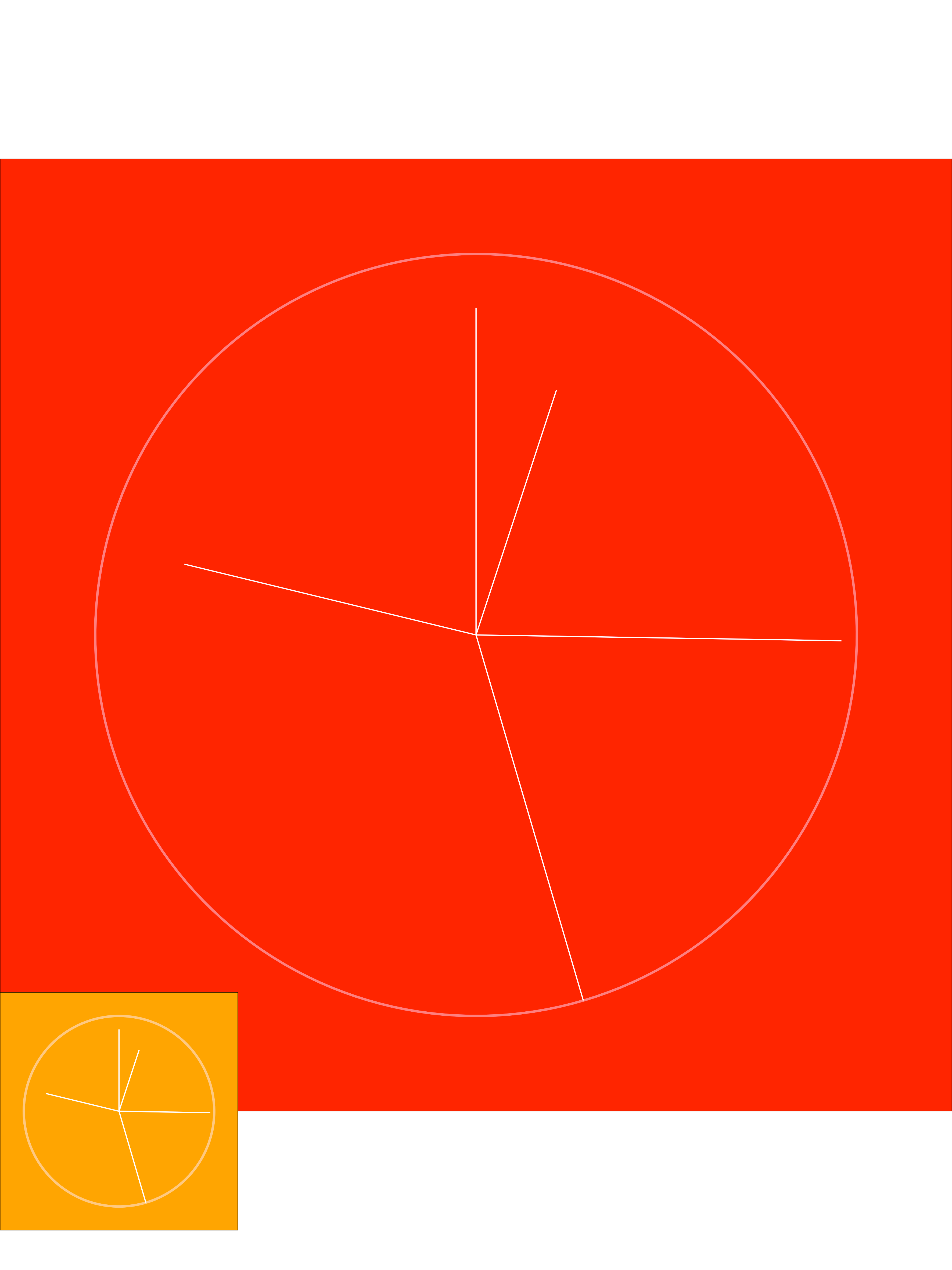# Sizing and positioning ggplots in cowplot

The code below has a main plot and then an inset plot. I'd like it to be portrait, so longer than it is wide. However, as the main plot is square, it shows an area that is not filled by the red rectangle.

In addition, the cowplot positioning for the inset should be 0 up from the bottom but is is indented upwards (there is a gap of white below the orange inset) and I'm not sure why.``````library(tidyverse)
library(cowplot)

foreColour <- "white"
backColour <- "red"
otherColour <- "orange"
theme_special <- theme_void() +
theme(axis.text = element_blank(),
axis.title = element_blank(),
legend.position = "none",
panel.grid = element_blank(),
plot.background = element_rect(fill = backColour),
plot.margin = unit(rep(0,4), "cm")
)

data <- data.frame(x = c(5, 25, 45, 78, 99),
y = c(67, 95, 99, 78, 85))
g <-
ggplot(data) +
theme_special +
coord_polar() +
xlim(c(0, max(data\$x))) +
ylim(c(0, max(data\$y))) +
#plot lines
geom_segment(aes(x = x, xend = x,
y = 0,
yend = y),
colour = foreColour,
size = 0.5, alpha = 1) +
#plot rim
geom_segment(aes(x = 0, xend = max(x),
y = max(y), yend = max(y)),
colour = "white", alpha = 0.1, size = 1)

gLegend <- g +
# Make background transparent as otherwise border shows
#theme(plot.background = element_blank())
# But for this test, make it another colour so we can see what's goin on
theme(plot.background = element_rect(fill = otherColour))

cowplot::ggdraw(g) +
draw_plot(gLegend, 0.0, 0.00, 0.25, 0.25)

ggsave("SO Cowplot Positioning.png",
device = "png",
scale = 1, width = 30, height = 40, units = "cm",
dpi = 300)
``````

### Content Attribution

This content was originally published by Chris at Recent Questions - Stack Overflow, and is syndicated here via their RSS feed. You can read the original post over there.